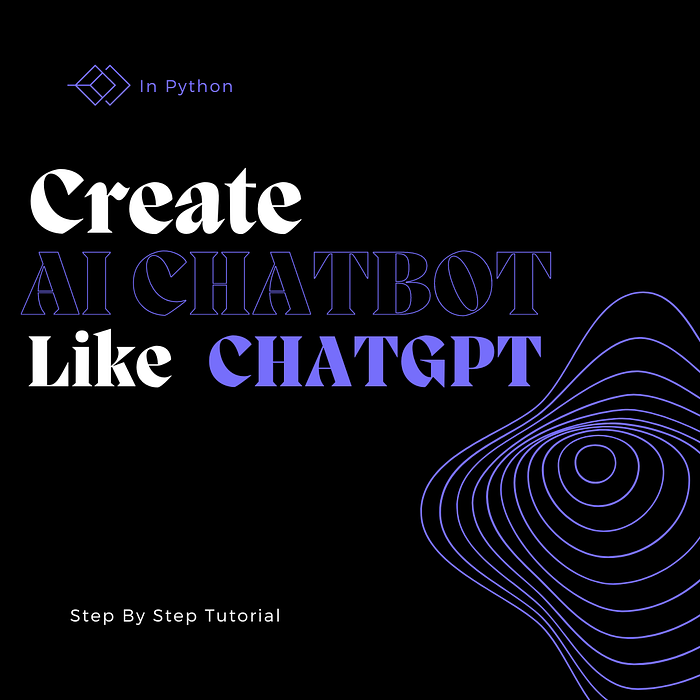# Step-by-Step Guide to Creating an AI Chatbot like ChatGPT

## Building an AI like ChatGPT is a complex process that requires a lot of expertise in various fields like natural language processing, deep learning, and machine learning. While this tutorial cannot…Building an AI like ChatGPT is a complex process that requires a lot of expertise in various fields like natural language processing, deep learning, and machine learning. While this tutorial cannot cover all the nuances of creating a system like ChatGPT, it will provide you with a step-by-step guide to building an AI-powered chatbot in Python that can understand and respond to natural language input. Before we get started, here are some prerequisites for building an AI like ChatGPT:

• Basic knowledge of Python programming
• Familiarity with machine learning and deep learning concepts
• Understanding of natural language processing

### Step 1: Install Required Libraries

To get started, we need to install the required Python libraries for our project. Here are the libraries we need:

• TensorFlow
• Keras
• Natural Language Toolkit (NLTK)
• Scikit-learn
• NumPy
• Pandas You can install these libraries using pip, the Python package manager. Run the following command in your terminal:
``````pip install tensorflow keras nltk scikit-learn numpy pandas
``````

### Step 2: Gather Training Data

The next step is to gather training data for our chatbot. This data will be used to train our machine learning model. You can use any data source for this, such as social media conversations, customer support chat logs, or any other text data that you have access to. In this tutorial, we will be using the Cornell Movie Dialogs Corpus, which is a dataset of conversations from movie scripts. You can download this dataset from the following link: http://www.cs.cornell.edu/~cristian/Cornell%5FMovie-Dialogs%5FCorpus.html

### Step 3: Preprocess the Data

Once you have your data, you need to preprocess it to make it suitable for machine learning. This involves cleaning the data, tokenizing it, and converting it into a format that our machine learning model can understand. Here are the steps to preprocess your data:

• Load the data into a Pandas dataframe
• Clean the text data by removing any unwanted characters, symbols, or punctuation marks
• Tokenize the text data into individual words or phrases
• Convert the text data into a numerical format that can be used for machine learning You can use NLTK to perform the text preprocessing. Here is some sample code to perform text preprocessing:
``````import nltk
from nltk.tokenize import word_tokenize

# Load data into a Pandas dataframe

# Clean data by removing unwanted characters, symbols, and punctuation marks
data['text'] = data['text'].str.replace('[^a-zA-Z0-9\s]', '')

# Tokenize text data into individual words
data['text'] = data['text'].apply(lambda x: word_tokenize(x))

# Convert text data into numerical format using one-hot encoding
from keras.preprocessing.text import Tokenizer
from keras.utils import to_categorical

tokenizer = Tokenizer()
tokenizer.fit_on_texts(data['text'])

# Convert text data into sequences of integers
sequences = tokenizer.texts_to_sequences(data['text'])

# Convert sequences into a matrix of one-hot vectors
one_hot_matrix = to_categorical(sequences)
``````

### Step 4: Build the Model

Once you have your preprocessed data, you can start building your machine learning model. For our chatbot, we will be using a deep learning model called a Seq2Seq model. Here is a sample code to build a Seq2Seq model in Keras:

``````from keras.layers import Input, LSTM, Dense
from keras.models import Model

# Define the model architecture
encoder_inputs = Input(shape=(None, input_dim))
encoder = LSTM(hidden_dim, dropout=0.2, return_state=True)
encoder_outputs, state_h, state_c = encoder(encoder_inputs)

decoder_inputs = Input(shape=(None, output_dim))
decoder = LSTM(hidden_dim, dropout=0.2, return_sequences=True, return_state=True)
decoder_outputs, _, _ = decoder(decoder_inputs, initial_state=[state_h, state_c])

dense = Dense(output_dim, activation='softmax')
output = dense(decoder_outputs)

model = Model([encoder_inputs, decoder_inputs], output)

#Compile the model
model.compile(optimizer='rmsprop', loss='categorical_crossentropy', metrics=['accuracy'])
``````

### Step 5: Train the Model

Once you have built your model, you can train it on your preprocessed data. You can use the fit method in Keras to train your model. Here is some sample code to train your model:

``````# Train the model
model.fit([encoder_input_data, decoder_input_data], decoder_target_data, batch_size=batch_size, epochs=epochs, validation_split=0.2)
``````

### Step 6: Test the Model

Once your model is trained, you can test it by providing some sample inputs and seeing how it responds. Here is some sample code to test your model:

``````# Define the encoder model to get the initial states
encoder_model = Model(encoder_inputs, [state_h, state_c])

# Define the decoder model to get the output sequence
decoder_state_input_h = Input(shape=(hidden_dim,))
decoder_state_input_c = Input(shape=(hidden_dim,))
decoder_states_inputs = [decoder_state_input_h, decoder_state_input_c]
decoder_outputs, state_h, state_c = decoder(decoder_inputs, initial_state=decoder_states_inputs)
decoder_states = [state_h, state_c]
decoder_outputs = dense(decoder_outputs)
decoder_model = Model([decoder_inputs] + decoder_states_inputs, [decoder_outputs] + decoder_states)

# Generate a response for a given input sequence
def generate_response(input_sequence):
input_sequence = input_sequence.reshape(1, input_sequence.shape, input_sequence.shape)
initial_states = encoder_model.predict(input_sequence)
target_sequence = np.zeros((1, 1, output_dim))
target_sequence[0, 0, target_token_index['<start>']] = 1
stop_condition = False
decoded_sequence = ''
while not stop_condition:
output_tokens, h, c = decoder_model.predict([target_sequence] + initial_states)
sampled_token_index = np.argmax(output_tokens[0, -1, :])
sampled_token = reverse_target_token_index[sampled_token_index]
decoded_sequence += ' ' + sampled_token
if (sampled_token == '<end>' or len(decoded_sequence) > max_decoder_seq_length):
stop_condition = True
target_sequence = np.zeros((1, 1, output_dim))
target_sequence[0, 0, sampled_token_index] = 1
initial_states = [h, c]
return decoded_sequence
``````

### Finally

Building an AI like ChatGPT requires a lot of expertise and knowledge of natural language processing, machine learning, and deep learning. However, this tutorial provides you with a basic understanding of how to build a simple chatbot using Python and Keras. With some additional experimentation and refinement, you can use these concepts to create a more sophisticated chatbot that can understand and respond to natural language input.